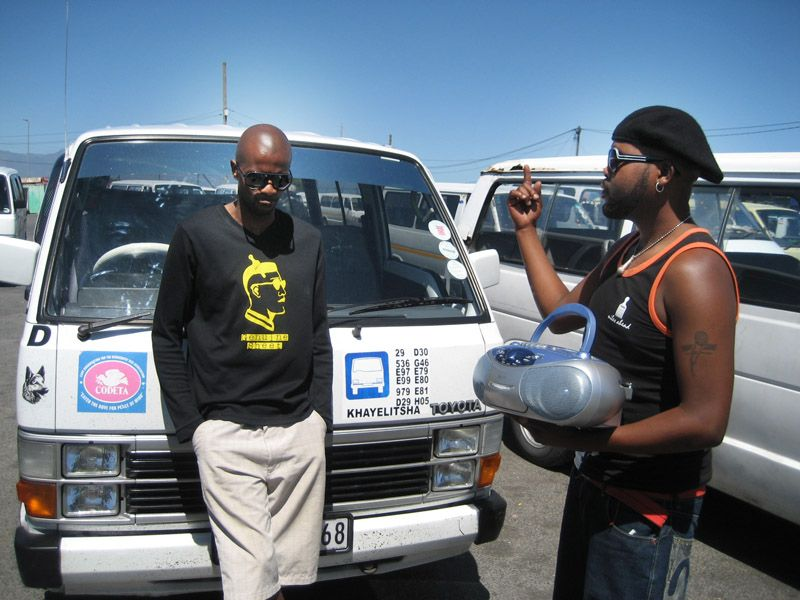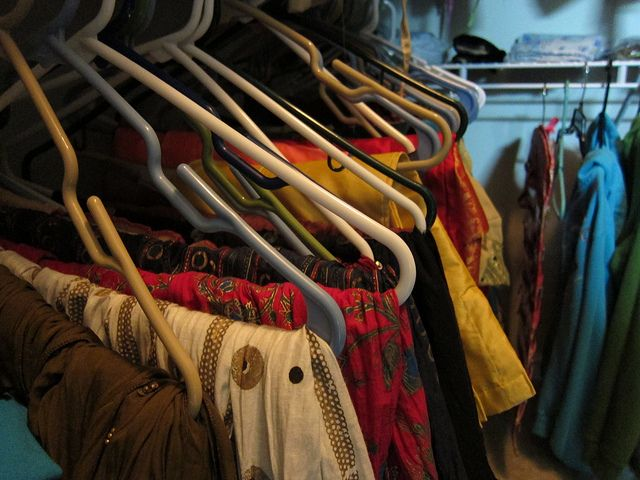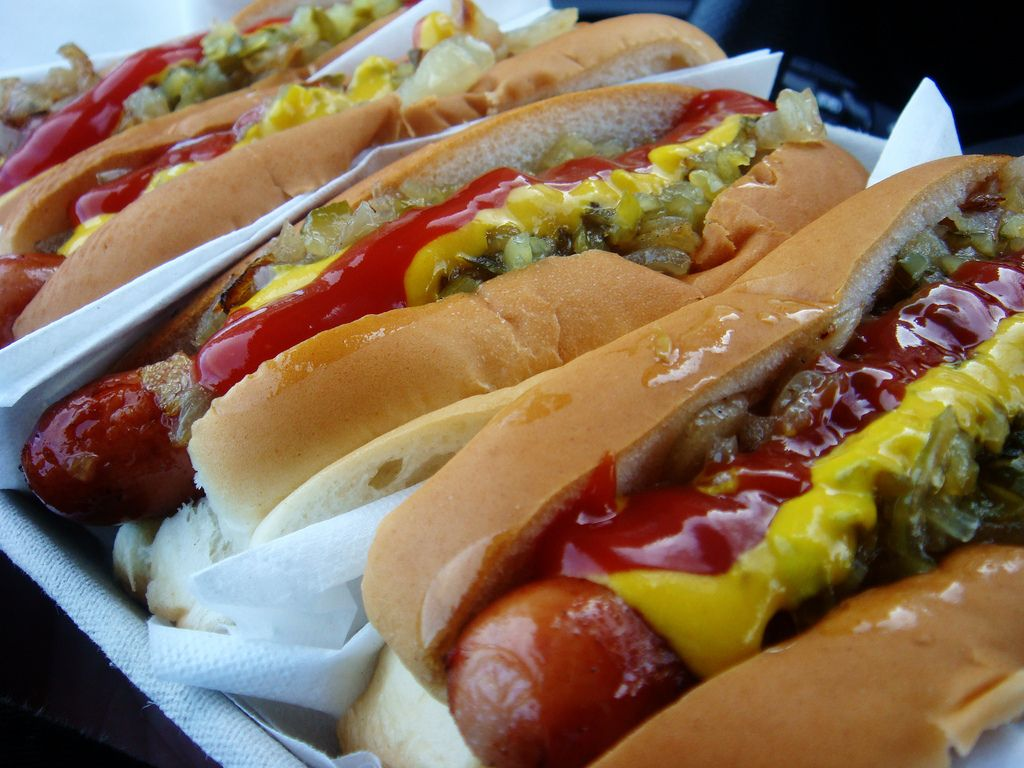Home Practice
For learners and parents For teachers and schools
Textbooks
Full catalogue
Pricing SupportLog in

We think you are located in United States. Is this correct?

# End of chapter activity

Exercise 8.6

Chuma writes down the following percentages for each of the items in her budget:

 Clothes $$\text{40}\%$$ Entertainment$$\text{30}\%$$ Fixed savings account $$\text{10}\%$$ Transport$$\text{5}\%$$ Donations $$\text{5}\%$$ Tuck shop spending $$\text{10}\%$$ Total $$\text{100}\%$$

If she has earned an income of $$\text{R}\,\text{500}$$ in a particular month, calculate exactly how much money she can allocate to each of the above items.

Clothes: $$\text{R}\,\text{200}$$, Entertainment: $$\text{R}\,\text{150}$$. Fixed savings account: $$\text{R}\,\text{50}$$. Transport: $$\text{R}\,\text{25}$$. Donations: $$\text{R}\,\text{25}$$. Tuck shop spending: $$\text{R}\,\text{50}$$.

Amanda budgeted her monthly expenses as follows:

 Clothes$$\text{25}\%$$ Entertainment $$\text{40}\%$$ Transport $$\text{10}\%$$ Tuck shop spending$$\text{15}\%$$ Donations $$\text{5}\%$$ Unforeseen costs $$\text{5}\%$$ Total $$\text{100}\%$$

If she has $$\text{R}\,\text{1 800}$$ to spend this month, how much money can she allocate to each expenditure item?

Clothes: $$\text{R}\,\text{450}$$. Entertainment: $$\text{R}\,\text{720}$$. Transport: $$\text{R}\,\text{180}$$. Tuckshop spending: $$\text{R}\,\text{270}$$. Donations: $$\text{R}\,\text{90}$$. Unforeseen costs: $$\text{R}\,\text{90}$$.

Look at the family budget for the month of December 2013, for the Philander family. There are two adults and two children (both in school) in the family.

 Item Expenditure Income Total income less total cost Fixed Variable Mrs Philander's salary $$\text{R}\,\text{9 500}$$ Mr Philander's salary a) Additional income b) Bond repayment c) Food d) Edgars clothing account payment e) School fees f) Transport g) Entertainment h) Savings i) Car repayment $$\text{R}\,\text{1 300}$$ Municipality rates j) Electricity $$\text{R}\,\text{200}$$ k) Vodacom contract cost l) i. l) ii. Telkom account m) i. m) ii. Total ? ? ? ? Surplus or deficit? ?

Complete the above budget of the family by calculating the following:

Mr Philander's income: He works $$\text{20}$$ days per month at a rate of $$\text{R}\,\text{500}$$ per day.

$$\text{20}$$ $$\times$$ $$\text{R}\,\text{500}$$ = $$\text{R}\,\text{10 000}$$

Additional income: Mr Philander owns additional property which he hires out to people at a fixed charge of $$\text{R}\,\text{2 500}$$ per month.

$$\text{R}\,\text{2 500}$$

The monthly bond repayments are fixed at $$\text{R}\,\text{5 550}$$ per month.

$$\text{R}\,\text{5 500}$$

The average amount spent on food each month comes to $$\text{R}\,\text{2 500}$$. Mrs Philander believes that this should be increased by $$\text{10}\%$$ due to recent food price increases.$$\text{R}\,\text{2 500}$$ + $$\text{R}\,\text{250}$$ = $$\text{R}\,\text{2 750}$$

Mr Philander pays Edgars an amount of $$\text{R}\,\text{800}$$ per month,. However, since he bought his children their school uniforms on account, he estimates that this amount will increase by a further $$\text{12}\%$$.

$$\text{R}\,\text{800}$$ + $$\text{R}\,\text{96}$$ = $$\text{R}\,\text{896}$$

The school fees are $$\text{R}\,\text{1 200}$$ per child per month.

$$\text{R}\,\text{1 200}$$ $$\times$$ $$\text{2}$$ = $$\text{R}\,\text{2 400}$$

Transport costs are as follows: For the children: taxi fare per child = $$\text{R}\,\text{5,00}$$ per trip to school and another $$\text{R}\,\text{5,00}$$ each for the trip home. There are $$\text{20}$$ school days in a month. Mr Philander first drives his wife to work and then goes to work himself. In the evenings he would pick her up and then drive home again. They both work $$\text{20}$$ days per month. Mr Philander has noticed that his car uses an average of $$\text{4}$$ litres of petrol per day each time he does this. On the other $$\text{10}$$ days of the month, his car uses an average of $$\text{3}$$ litres per day. The cost of petrol is $$\text{R}\,\text{10,50}$$ per litre. Calculate the total amount that should be budgeted for transport.Taxi fare: $$\text{R}\,\text{10}$$ per day $$\times$$ $$\text{2}$$ children $$\times$$ $$\text{20}$$ days = $$\text{R}\,\text{400}$$. Petrol: ($$\text{20}$$ $$\times$$ $$\text{4}$$ litres $$\times$$ $$\text{R}\,\text{10,50}$$) + ($$\text{10}$$ $$\times$$ $$\text{3}$$ litres $$\times$$ $$\text{R}\,\text{10,50}$$) = $$\text{R}\,\text{840}$$ + $$\text{R}\,\text{315}$$ = $$\text{R}\,\text{1 155}$$

The amount budgeted for entertainment is estimated at $$\text{5}\%$$ of the combined income of Mr and Mrs Philander.

Total salaries = $$\text{R}\,\text{19 500}$$. $$\text{5}\%$$ of this is $$\text{R}\,\text{975}$$.

Savings are currently $$\text{5}\%$$ of Mrs Philander's income.

$$\text{5}\%$$ of $$\text{R}\,\text{9 500}$$ = $$\text{R}\,\text{475}$$.

The amount budgeted for municipal rates is $$\text{5}\%$$ of the total income earned by the Philander household.

Total income = salaries + additional income = $$\text{R}\,\text{19 500}$$ +$$\text{R}\,\text{2 500}$$ = $$\text{R}\,\text{22 000}$$. $$\text{5}\%$$ of this is $$\text{R}\,\text{1 100}$$.

The fixed component of the electricity account is currently $$\text{R}\,\text{200}$$ per month. The variable component is calculated as follows:The average amount of electricity consumed by the Philander household is $$\text{550}$$ kilowatt hours per month at a rate of $$\text{R}\,\text{0,50}$$ per kilowatt hour.

($$\text{550}$$ $$\times$$ $$\text{R}\,\text{0,50}$$) =$$\text{R}\,\text{275}$$

Vodacom contract cell phone account:

Fixed component: $$\text{R}\,\text{135}$$ per month

$$\text{R}\,\text{135}$$

Variable component: $$\text{R}\,\text{0,80}$$ per minute of airtime used during peak time. An average of $$\text{100}$$ minutes of airtime per month is used during peak time. Off peak minutes are charged at a rate of $$\text{R}\,\text{0,40}$$ per minute. An average of $$\text{200}$$ minutes per month is used during this time.

($$\text{100}$$ $$\times$$ $$\text{R}\,\text{0,80}$$) + ($$\text{200}$$ $$\times$$ $$\text{R}\,\text{0,40}$$) = $$\text{R}\,\text{160}$$

Telkom account:

Fixed component is $$\text{R}\,\text{400}$$ per month.

$$\text{R}\,\text{400}$$

Variable component: $$\text{R}\,\text{0,50}$$ per minute during normal time. An average of $$\text{350}$$ minutes is spent each month on the phone during this time. Call more time is calculated at $$\text{R}\,\text{7}$$ per night. The children use the phone an average of $$\text{20}$$ nights per month during this time.($$\text{350}$$ $$\times$$ $$\text{R}\,\text{0,50}$$) + ($$\text{R}\,\text{7}$$ $$\times$$ $$\text{20}$$) = $$\text{R}\,\text{315}$$.The total for fixed expenses is $$\text{R}\,\text{12 456}$$. The total for variable expenses is $$\text{R}\,\text{5 630}$$. So the total for all expenses is $$\text{R}\,\text{18 086}$$. The total income for the household is $$\text{R}\,\text{22 000}$$, so yes - they are within budget, because their income is greater than their total expenditure and they have a surplus of money.